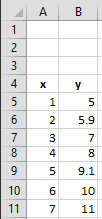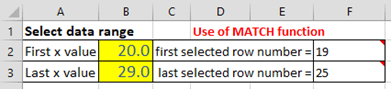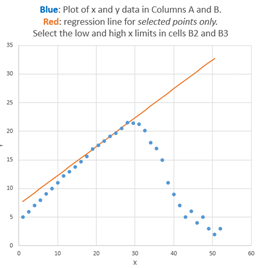## Z. Dealing with variable data arrays in spreadsheets

When applying spreadsheet templates of the type described in this book to your own data, it's often necessary to modify the templates to accommodate different numbers of data points or of components. This can be tedious to do, especially because you need to remember the syntax of each of the spreadsheet functions that you want to modify. This section describes ways to construct spreadsheets that automatically adapt to different data sets, without your taking the time and effort to modify the spreadsheet formulas for each case. This involves employing some less commonly used built-in functions in Excel or OpenOffice Calc, such as MATCH, INDIRECT, COUNT, IF, and AND.

The MATCH function. In signal processing using spreadsheets, it's common to have x-y arrays of data of variable length, such as spectra (x=wavelength, y=absorbance or intensity) or chromatograms (x=time, y=detector response). Forexample, consider this small array of x and y values pictured in the spreadsheet fragment on the left. Spreadsheet formulas normally refer to cells by their row and column address, but for an x-y data set like this, it's more natural to refer to a data point by its independent variable x, rather than its row and column address. For example, suppose you want to select the data point where x=2, irrespective of what cells they inhabit. You can do that with the MATCH function. For example, if you set cell B2 to the desired x value (2), then the cell formula MATCH(B2,A5:A11) + ROW(A5) will return the row number of that point, which is 6 in this case. Later, if you were to move or expand this table, by dragging it or by inserting or deleting rows or columns, the spreadsheet will automatically adjust the MATCH function to compensate, returning the new row number of the requested point.

The INDIRECT function. The usual way to reference the value in a cell is to specify its row and column address. For example, take the small array of x and y values pictured above. To refer to the contents of column B, row 6, you could write "=B6", which in this case will evaluate to 5.9. This is referred to as "direct" addressing. In contrast, to use "indirect" addressing you can write "=INDIRECT("B"&A1)", then put the number "6" in cell A1. The "&" character is simply "glue" that joins "B" to the contents of A1, so in that case "B"&A1 evaluates to "B6" and the result is the same as before: the contents of cell B6, which is 5.9. However, if you change cell A1 to 9, then "B"&A1 would evaluate to "B9", and the result would be the contents of cell B9, which is 9.1. In other words, the indirect function allows the addresses of cells to be calculated within the spreadsheet rather than being typed in as a fixed number. This makes it possible for spreadsheets to adjust their own addresses based on a calculated result, for example to adapt their calculations to fit the number of data points in that particular data set.

These examples were done in what is called the "A1" reference style, where the columns are referred to by letters; it's also possible to use the "R1C1" reference style, where both the and the rows columns are referred to by numbers. For example =INDIRECT("R"&A2&"C"&A1,FALSE)", with the row number in A2 and the columns number in A1. (The "FALSE" just means that the "R1C1" reference style is used).
The "R1C1" reference style allows both the row and column address to be expressed as numbers that can be calculated within the spreadsheet.

You can use the same technique to compute ranges of cell addresses. For example, you could compute the sum of the y values in column B, rows 5 to 11, by writing SUM(B5 : B11) by direct addressing. But suppose you wanted to compute the sum of all the numbers in column B between a variable first row and variable last row. If you put the first row number in A1 and the last row number in row A2, the address of the first cell would be "B"&A1 and the address of the last cell would be "B"&A2. So you would form the range of cell addresses by using "&" to glue together those two addresses, with a colon in-between ("B"&A1&" : B"&A2). The sum would be SUM(INDIRECT("B"&A1&" : B"&A2)), which is 56. Yes, it's longer, but the advantage over direct addressing is that you can adjust the range by changing just two cells rather that retyping the formula. It's the same for other functions that need a range of cells, such as AVERAGE, MAX, MIN, STDEV, etc. For examples of its use in signal processing, see
VariableSmooth.xlsx (screen image).

For functions that require two ranges, separated by a comma, you can use the same technique. For example. suppose you want to compute the slope of the linear regression line between the x values in column A and the y values in column B in the spreadsheet except in the previous figure, using the built-in SLOPE function. SLOPE requires two ranges, first the dependent (y) values and them the independent x values. By direct addressing, the slope is SLOPE(B5 : B11,A5 : A11) . By indirect addressing, you need two separate "indirect" functions, one for each range, separated by a comma. Here's what it looks like all together: SLOPE(INDIRECT("B"&A1&" : B"&A2), INDIRECT("A"&A1&" : A"&A2)), where the x values are in column A, the y values in column B, and the first and last row numbers are in cells A1 and A2 respectively. It works exactly the same for the two related functions that calculate the INTERCEPT and RSQ (the R2 value) of the regression line. I admit that the formula is confusing to read at first, but it works. Just break it down into its parts.

A working example. An example of the use of the MATCH and INDIRECT functions working together is demonstrated in the spreadsheet "SpecialFunctions.xlsx" (
Graphic), which has a larger table of x-y data stored in columns A and B, starting in row 7. The idea here is that you can select a limited range of x values to work with by typing in the lowest x and the highest x value in cells B2 and B3, the two cells with ayellow background, shown on the left. The spreadsheet uses the MATCH functions in cells F2 and F3 to compute the corresponding row numbers, which are then used in the INDIRECT functions in the "Properties of selected data range" section to compute the maximum, average, and average of x and of y, and also the slope, intercept, and R2 values of the y vs x linear regression line over that selected x interval. The regression line, fitting only the data from x=20 to 29, is shown in red in the graph on the right, superimposed on the complete data set (blue dots). By simply changing thex-axis limits in cells B2 and B3, the spreadsheet and the graph re-calculates, without your having to edit any of the cell formulas. Try it yourself. (Hint: Cells with red mark in upper right corner contain helpful pop-up notes: you can float the mouse pointer over any such cell to reveal its cell formula and/or an explanation).

Columns J and K of this sheet also show how to use the "IF" and "AND" functions to copy data from columns A and B into columns J and K only those data points that fall between the two specific x limits.

If desired, you can add more data to the end of columns A and B, limited only be the range of the match functions in cells F2 and F3 (which are initially set to 1000, but that could be as large as you need). The total number of numerical values in the data set is computed in cell I15, using the "COUNT" function (which, as the name suggests, counts the number of cells in a range that contains numbers and does not count empty cells or cells with letters).

Measuring peak location. A common signal processing operation is finding the x-axis value where the y-axis value is maximum. This can be broken down into four steps:

(1) Define the range of values, either directly or using the indirect function, e.g. INDIRECT("B"&F2&":B"&F3)
(2) Determine the maximum y value in that range with the
MAX function, e.g.:  MAX(INDIRECT("B"&F2&":B"&F3))
(3) Determine the row number in which that number appears
with the MATCH function, e.g.MATCH(H20,B7:B1000,0)+ROW(A6)
(4) Determine the value of x in that row with the INDIRECT function,
e.g.
INDIRECT("A"&H21)

Each step references the results of the one before it. These steps are illustrated in the same "" spreadsheet in column H, rows 20-23. The result is that the maximum y (21.5) occurs at x=28. These steps can even be combined into one long formula (cell H23), although this is harder to read, and harder to document, than the formulas for the separate steps. The peak finder spreadsheet uses this technique.

The LINEST function. Indirect addressing is particularly useful when using array functions such as LINEST or the matrix algebra functions. The demonstration spreadsheet "IndirectLINEST.xlsx" (graphic link) shows how this works for the multiwavelength spectroscopy analysis of a mixture of three overlapping components by the CLS method. The measured mixture spectrum is in column C, rows 29-99 and the spectra of the three pure components are in columns D, E, and F. Cell C12 "=COUNT(C29:C1032)" counts the number of rows of data (i.e. number of wavelengths) in column C starting at row 29, and cell G3 counts the number of components (in this case 3). These are used to determine the first and last row and column for the indirect addresses in LINEST in cell C17. The measured peaks heights calculated by LINEST for the three peaks are given in row 17, columns C, D, can E, and the predicted standard deviations are in the row below. In this spreadsheet the data are actually simulated (over in columns O - U), so the true peaks heights are known and therefore the absolute accuracy can be calculated (row 26, C, D, and E) and compared to the predicted standard deviations. Press the F9 key to recalculate with an independent noise sample, which is equivalent to taking another measurement of the same sample. Because of the use of INDIRECT addressing, you can add or subtract data points at the end of columns C - E and the calculations work with no other changes. Examples of its use in signal processing are on CurveFittingB.html#spreadsheets.

This page is part of "A Pragmatic Introduction to Signal Processing", created and maintained by Prof. Tom O'Haver , Department of Chemistry and Biochemistry, The University of Maryland at College Park. Comments, suggestions and questions should be directed to Prof. O'Haver at toh@umd.edu. Updated July, 2022.